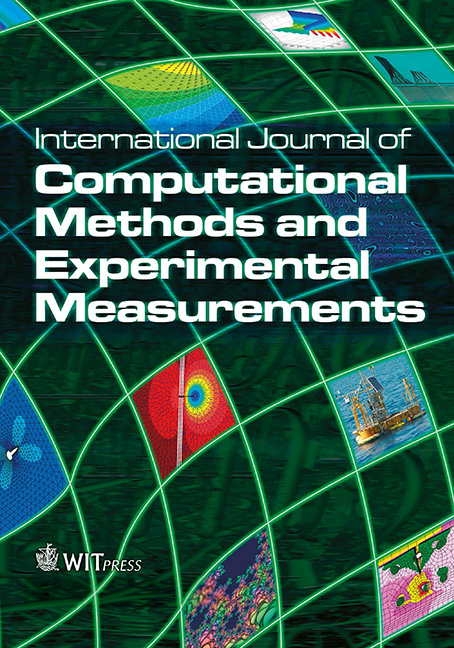WIT Press

35 years of advancements with the complex variable boundary element method

Price

Free (open access)Volume

Volume 7 (2019), Issue 1

Pages

12

Page Range

1 - 13

Paper DOI

10.2495/CMEM-V7-N1-1-13

WIT Press

Author(s)

Noah J. Demoes, Gabriel T. Bann, Bryce D. Wilkins, Theodore V. Hromadka II, & Randy Boucher

Abstract

The Complex Variable Boundary Element Method, or CVBEM, was first published in Journal of Numerical Methods in Engineering in year 1984 by authors Hromadka and Guymon . Since that time, several papers and books have been published that present various aspects of the numerical technique as well as advances in the computational method such as extension to three or higher dimensions for arbitrary geometries, nonhomogeneous domains, extension to use of a Hilbert Space setting as well as collocation methods, inclusion of the time derivative via coupling to generalized Fourier series techniques, examination of various families of basis functions including complex monomials, the product of complex polynomials with complex logarithm functions (i.e., the usual CVBEM basis functions), Laurent series expansions, reciprocal of complex monomials, other complex variable analytic functions including exponential and others, as well as linear combinations of these families. Other topics studied and developed include rotation of complex logarithm branch-cuts for extension of the problem compu- tational domain to the exterior of the problem geometry, depiction of computational error in achieving problem boundary conditions by means of the approximate boundary technique, mixed boundary value problems, flow net development and visualization, display of flow field trajectory vectors in two and three dimensions for use in depicting streamlines and flow paths, among other topics. The CVBEM approach has also been extended to solving partial differential equations such as Laplace’s equation, Poisson’s equation, unsteady flow equation, and the wave equation, among other formulations that include sources, sinks and combinations of these equations with mixed boundary conditions.

In the current paper, a detailed examination is made of the performance between four families of basis functions in order to assess computational efficiency in problem solving of two dimensional potential problems in a high aspect ratio geometric problem domain. Two selected problems are presented as case studies to demonstrate the different levels of success by each of the four families of examined basis functions. All four families involve basis functions that solve the governing partial differential equation, leaving only the goodness of fit in matching boundary conditions of the boundary value problem as the computational optimization goal. The modeling technique is implemented in com- puter programs Mathematica and MATLAB. Recommendations are made for future research directions and lessons learned from the current study effort.

Keywords

complex variables, complex variable boundary element method, CV BEM, basis functions, ideal fluid flow, Laplace’ s equation